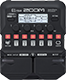# AkustMNEM

Discussion in 'Zoom G1/G1X Four' started by Ikan, Jul 23, 2022.

1. AkustMNEMDevice: Zoom G1 Four
Firmware: 2.00

Name on device: Nothing EM
Optimized for: Guitar Amp

Effects chain:M N E M

Effect: "Comp" (Dynamics), active - "yes"
"Sense" = 1
"Attack" = Fast
"Tone" = 0
"Volume" = 90

Effect: "Aco.Sim" (Overdrive / Distortion), active - "yes"
"Top" = 100
"Body" = 100
"Tone" = 100
"Volume" = 80

Effect: "Chorus" (Modulation), active - "yes"
"Depth" = 20
"Rate" = 25
"Tone" = 7
"Mix" = 50

Effect: "Hall" (Reverb), active - "yes"
"PreD" = 50
"Decay" = 10
"Mix" = 70
"Tail" = On

Effect: "Gt GEQ 7" (Filter), active - "yes"
"100" = 3.0
"200" = 0.5
"400" = -5.0
"800" = -2.5
"1.6k" = -5.5
"3.2k" = 6.5
"6.4k" = 8.0
"VOL" = 80

Patch Volume: 50